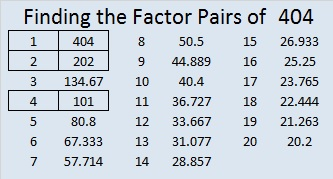# 404 and Level 2

It may seem like trivial trivia, but every factor of 404 is a palindrome. Scroll down past the puzzle to find out what all those factors are.Print the puzzles or type the factors on this excel file: 12 Factors 2015-02-23

• 404 is a composite number.
• Prime factorization: 404 = 2 x 2 x 101, which can be written 404 = (2^2) x 101
• The exponents in the prime factorization are 2 and 1. Adding one to each and multiplying we get (2 + 1)(1 + 1) = 3 x 2  = 6. Therefore 404 has exactly 6 factors.
• Factors of 404: 1, 2, 4, 101, 202, 404
• Factor pairs: 404 = 1 x 404, 2 x 202, or 4 x 101
• Taking the factor pair with the largest square number factor, we get √404 = (√4)(√101) = 2√101 ≈ 20.0998This site uses Akismet to reduce spam. Learn how your comment data is processed.